# A Study in Sphere Packing

by Kirby Urner
First posted: October 29, 1997
Last updated: October 29, 1997

We learned in An Introduction to Quadrays that the six edges of the quadrays' framing tetrahedron, doubled to give positive and negative directionality, comprise the 12 directions from the center of a cuboctahedron to its 12 vertices.

Put another way, the back and forth movements along the six edges of a regular tetrahedron provide the 12 stepwise jumps available between neighboring unit-radius sphere centers in the isotropic vector matrix. Packing outwardly in layers from a nuclear sphere, this packing takes on a cuboctahedral conformation.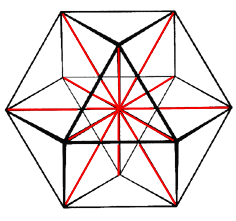The goal of this paper is to leverage our investment in quadrays to get some renderings of cuboctahedral sphere packings at different frequencies. Our investment so far consists of some computer source code defining a 4D turtle class. The turtle moves around in space in response to either quadray or xyz inputs.

The 12 directions from a nuclear sphere center to the centers of the 12 spheres packed around it are defined by the following quadray coordinates:

(0,1,1,2) (0,1,2,1) (0,2,1,1)
(1,0,1,2) (1,0,2,1) (1,1,0,2)
(1,1,2,0) (1,2,0,1) (1,2,1,0)
(2,0,1,1) (2,1,0,1) (2,1,1,0)

Notice that specifying the position of the 2 and the 0 unambiguously identifies these 12 quadrays, the remaining coordinates being both 1. If we say the 2nd coordinate is 2, and the 4th is 0, then we know (1,2,1,0) is the quadray meant.

With four positions (or slots) for our tokens, 2 and 0, we have 12 possibilities. The matrix at right shows all possible position pairs, but since our tokens never share the same position, the position pairs (1,1) (2,2) (3,3) and (4,4) are out of bounds. That leaves 12 possibilities -- all remaining pairs once the four along the diagonal are removed.

Note that if both tokens were the same number, like 1 and 1, we would have only six unique possibilities. The diagonal would drop out as before, plus those above the diagonal are merely position pairs with the tokens reversed -- and in the case where both tokens are the same, these mirror pairs would be identical.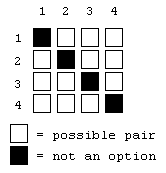Given the above reasoning, we can write code to generate the 12 quadrays. We start by initializing all four slots to 1, and then traverse the possible pairs, changing one slot to 2, and the other to 0 -- but skipping the black diagonal possibilities where row = column (i = j). Here's the code: ``` procedure defineVE local i,j,k k=1 && goes from 1 to 12 for i = 1 to 4 && row for j = 1 to 4 && column if i=j && skip if row = column loop endif this.VEvector(k,1)=i && the position of the 2 this.VEvector(k,2)=j && the position of the 0 k=k+1 endfor endfor endproc ``` The figure at right was developed by cycling through all 12 quadrays and recording the resulting spatial locations. The locations were filed in a table of data points, via a filing procedure which screens for duplicates (only trully new locations are allowed into the table). Duplication is not a problem when filing the nucleus position (0,0,0,0) and then cycling through the 12 surrounding locations, but will become a problem below. The 13 total addresses were defined as the centers of unit-radius spheres and written out in a format understood by Povray, a public domain ray tracing and rendering package.Suppose we want a second layer of spheres around the first, then a third layer and so on. We will need to fill our data points table with all the unique sphere center locations. One method for accomplishing this, while not the most efficient by some measures, has the advantage of making economical use of code. Our basic loop involves starting at some home position and recording the locations of the 12 surrounding positions. Using a programming technique called recursion, we can make each of the 12 surrounding positions a new home position, mapping the next surrounding 12, and so on. But if one of the 12 surrounding spheres becomes a new home base, then the 12 spheres surrounding it will include one or more already recorded. This is an inefficiency and is also what requires compensating code to screen out all the duplicates generated by such a technique. The recursive code is provided below, where the parameter n controls how deeply the recursion drills before moving to the next location in the outermost loop. In this example, n was set to 3, for 3 frequency.
 ```procedure calcve(n,a,b,c,d) local k, q(4) for k = 1 to 12 q=1 q(this.VEvector(k,1))=2 q(this.VEvector(k,2))=0 q(1)=a+q(1) q(2)=b+q(2) q(3)=c+q(3) q(4)=d+q(4) this.makepoint; (q(1),q(2),q(3),q(4)) if n>1 this.calcVE; (n-1,q(1),q(2),q(3),q(4)) endif endfor endproc ```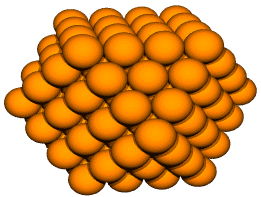The above algorithm is also inefficient in the sense that it computes the locations of all spheres in the packing, whereas clearly only a minority of these spheres are visible -- a lot more data is going to the ray tracing program than meets the eye. In case you are wondering why the letters VE keep recurring in the snippets of program code, you should know that a cuboctahedral wireframe of 24 circumferential and 24 radial vectors (the radial members are doubled -- think of eight hinge-bonded tetrahedra) is known as a vector equilibrium in synergetics. At 5-frequency, this recursive approach requires around 15 minutes on the author's 133 Mhz Pentium to compute the 1 + 12 + 42 + 92 + 162 + 252 sphere locations and write the Povray file. Note that the number of spheres in each successive layer is given by the formula: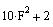And the cumulative number of spheres by: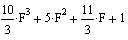A related study might replace the spheres with spokes and hubs, giving a more skeletal view of the 60 and 90 degree angles between IVM rods, which outline tetrahedra and octahedra of relative volume 1:4.
 For further reading: Source code for the packTurtle subclass used in this study Sphere packing equations Spheres of Relevance section of Synergetics in the 1990s: The Invention Behind the Inventions An Introduction to Quadrays Synergetics on the Web maintained by Kirby Urner